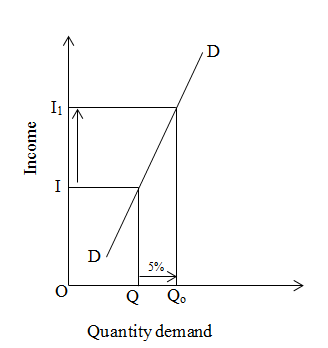# Income elasticity of demand less than one

If the percentage change in quantity demand is less than the percentage change in income is known as income elasticity of demand less than one.

For example, change in demand by 5% due to change in income by 10%.On the above figure, initial income is OI and quantity demand is OQ. When income increases from I to I1 by 10% then demand increases from Q to Q1 by 5%. This is known as elasticity of demand less than one.